# Maharashtra Board Class 11 Chemistry Important Questions Chapter 7 Modern Periodic Table

Balbharti Maharashtra State Board 11th Chemistry Important Questions Chapter 7 Modern Periodic Table Important Questions and Answers.

## Maharashtra State Board 11th Chemistry Important Questions Chapter 7 Modern Periodic Table

Question 1.
Mention features of Mendeleev’s periodic table.
Features of Mendeleev’s periodic table:

• In Mendeleev’s periodic table, all 63 elements were arranged in an increasing order of their atomic masses. The serial or ordinal number of an element in the increasing order of atomic mass was referred to as its atomic number.
• Mendeleev’s periodic table consisted of vertical groups and horizontal series (now called periods).
• Elements belonging to the same group showed similar properties.
• Properties of elements in a series/period showed gradual variation from left to right.

Question 2.
Why was Mendeleev’s periodic table readily accepted by scientific community?
Mendeleev’s periodic table was readily accepted by scientific community due to the following advantages:
i. Mendeleev had left some gaps corresponding to certain atomic numbers in the periodic table so as to maintain periodicity of the properties. When the elements corresponding to these atomic numbers were discovered, they fitted well into the gaps with their properties as predicted by Mendeleev’s periodic law.

ii. Mendeleev did not predicted presence of inert gases, however, they were discovered in later years. It was possible to accommodate inert gases in Mendeleev’s periodic table by creating an additional group without disturbing the position of other elements in his periodic table.

Question 3.
Give reason: Mendeleev’s periodic law was modified into modern periodic law.

• Henry Moseley in 1913, studied X-ray spectra of large number of elements.
• He observed that the frequency of X-ray emitted from an element is related to atomic number (Z) of an element and not its atomic mass.
• Therefore, the atomic number, Z, was considered as more fundamental property of the atom than the atomic mass.
• As a result, Mendeleev’s periodic law was modified.

Question 4.
Define atomic number.
Atomic number (Z) is the total number protons present in the atom of an element.Question 5.
State the modern periodic law.
Modern periodic law: “The physical and chemical properties of elements are a periodic function of their atomic numbers”.

Question 6.
Periods and groups present in the modern periodic table are numbered based on whose recommendation ?
Numbering of the periods and groups in the modem periodic table is based on the recommendation provided by the International Union of Pure and Applied Chemistry (IUPAC).

Question 7.
Write a note on: Structure of the modern periodic table.
Structure of the modern periodic table:
i. The modem periodic table also known as the Tong form of periodic table’ has number of boxes formed by the intersection of horizontal rows and vertical columns.
ii. The horizontal rows are called periods and the vertical columns are called groups.
iii. There are seven periods numbered from 1 to 7 and eighteen groups numbered from 1 to 18.
iv. There are total 118 boxes in the modem periodic table which are filled with 118 elements discovered till now including manmade elements.
v. The modem periodic table is divided into four blocks i.e., s-block, p-block, d-block and f-block.

• Two groups on the extreme left of the modem periodic table form the s-block.
• Six groups on the extreme right constitute the p-block.
• Ten groups in the centre form the d-block
• Two series at the bottom of the modem periodic table constitute the f-block. It contains fourteen elements in each series.

Question 8.
State the relationship between the modern periodic table and electronic configuration in periods.

• The modem periodic table is based on the atomic numbers of the elements. When elements are arranged in an increasing order of atomic number (Z), periodicity is observed in their electronic configurations which reflects in the characteristic structure of the modem periodic table.
• The location of elements in the modem periodic table is correlated to quantum numbers of the last filled orbital.
• Along a period, the atomic number increases by one and one electron is added to the outermost shell which forms neutral atom of the next element.
• The period number is same as the principal quantum number ‘n’ of the valence shell of the elements.
• A period begins with filling of a particular shell and ends when the valence shell attains complete octet configuration (or duplet, in case of the first period).
• The next period begins with addition of electron to the next shell of higher energy compared to the previous period. e. g. First shell of the elements gets filled along the first period while second shell starts filling in the second period and addition of electrons continues till second shell (valence shell) attains stable electronic configuration.Question 9.
Give reason: First period in the modern periodic table contains only two elements.

• Elements present in the first period i.e., H and He contain only one shell which is also their valence shell and can accommodate maximum two electrons.
• As first shell can accommodate only two electrons, first period ends at He which has a complete duplet. Hence, first period in the modem periodic table contains only two elements.

Question 10.
Write names and electronic configurations of elements of first period in the modern periodic table. Identify which of them has the stable complete electronic configuration.

• Hydrogen (H) : 1s2, Helium (He) : 2s2
• Since helium has a complete duplet i.e., two electrons in its valence shell, it has the stable complete electronic configuration.

Question 11.
Explain how does the filling of electrons takes place in the modern periodic table across:
i. Second period
ii. Third period
i. Filling of electrons in the second period:

• In the second period, electrons are filled in the second shell i.e., n = 2.
• This shell can accommodate a maximum of eight electrons and gets filled as the atomic number increases along the second period.
• The second period begins with Li (Z = 3): 1s2 2s1 and ends up with Ne (Z = 10): 1s2 2s2 2p6.
• Neon has complete octet with 8 electrons in its valence shell. Therefore, the second period contains eight elements.

ii. Filling of electrons in the third period:

• The third period corresponds to the filling of the third shell i.e. n = 3.
• The third period also contains eight elements.
• It begins with the filling of electrons in the first element Na (Z = 11) : [Ne] 3s1 and ends with the last element Ar (Z = 18) = [Ne] 3s2 3p6.
• The condensed electronic configurations for the elements of third period is [Ne] 3s1-2 3p1-6.

Question 12.
There are 18 elements in the fourth period of the modern periodic table. Explain.

• The fourth period corresponds to the filling of fourth shell, n = 4.
• Therefore, it begins with filling of 4s subshell. The first two elements of the fourth period are K (Z = 19) : [Ar] 4s1 and Ca (Z = 20) : [Ar] 4s2.
• According to the aufbau principle, the next higher energy subshell is 3d, which can accommodate up to 10 electrons. Thus, filling of the 3d subshell results in the next 10 elements of the fourth period i.e., from Sc (Z = 21) : [Ar] 4s23d1 to Zn (Z = 30): [Ar] 4s23d10.
• After filling of 3d subshell, the electrons enter the 4p subshell which can accommodate maximum 6 electrons. Hence, filling of 4p subshell results in the next 6 elements i.e., from Ga (Z = 31): [Ar] 4s23d10 4p1 to Kr (Z = 36): [Ar] 4s2 3d10 4p6.
• Thus, the elements in fourth period are: 2 elements (with 4s subshell), 10 elements (with 3d subshell) and 6 elements (with 4p subshell).
• Hence, there are 18 elements in the fourth period of the modem periodic table.Question 13.
Why does the fifth period of the modern periodic table contain 18 electrons?
The fifth period accommodates 18 elements as a result of successive filling of electrons in the 5s, 4d and 5p subshells.

Question 14.
What is the general trend followed while filling of electrons across a period in the modern periodic table.

• A period begins by filling of one electron to the ‘s’ subshell of a new shell and ends when an element corresponding to the same shell attains complete octet (or duplet).
• Between these two ‘s’ and ‘p’ subshell of the valence shell, the inner subshells ‘d’ and ‘f’ are filled successively following the aufbau principle.

Question 15.
What is the subshell in which the last electron of the first element in the 6th period enters?
The 6th period begins by filling the last electron in the shell with n = 6. The lowest energy subshell of any shell is ‘s’. Therefore, the last electron of the first element in the 6th period enters the subshell ‘6s’.

Question 16.
How many elements are present in the 6th period? Explain.

• The 6th period begins by filling the last electron in the subshell ‘6s’ and ends by completing the subshell ‘6p’. Therefore, the sixth period has the subshells filled in increasing order of energy as 6s < 4f < 5d < 6p.
• The electron capacities of these subshells are 2, 14, 10 and 6, respectively. Therefore, the total number of elements in the 6th period is 2 + 14 + 10 + 6 = 32.Question 17.
How does electronic configuration vary down a group in the modern periodic table?

• As we move from top to bottom in a group, a new shell gets added successively in the atom of an element. Therefore, the last electron enters in a new shell down the group.
• Hence, the general outer electronic configuration of the elements in a group remains the same. This holds true for groups 1, 2 and 3 elements.
• In the groups 13 to 18 the appropriate inner ‘d’ and ‘f’ subshells are completely filled and the general outer electronic configuration is the same down the groups 13 to 18.
• However, in the groups 4 to 12, the ‘d’ and ‘f subshells are introduced at a later stage (4th period for ‘d’ and 6th period for ‘f’) down the group. As a result, variation in the general outer configuration is introduced only at the

Note: General outer electronic configuration in groups 1 to 3 and 13 to 18.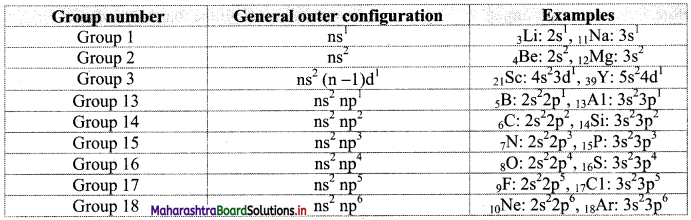Question 18.
On what basis is the modern periodic table divided into four blocks?
The modem periodic table is divided into four blocks based on the subshell in which the last electron enters.

Question 19.
Why elements of group 1 and group 2 are known as s-block elements?

• The subshell in which the last electron enters decides the block to which an element belongs.
• In group 1 and group 2 elements, the last electron is filled in the s subshell.

Therefore, the elements of group 1 and group 2 are known as s-block elements.

Question 20.
Elements belonging to which groups constitute the p-block and why?

• Elements belonging to groups 13, 14, 15, 16, 17 and 18 constitute the p-block.
• The last electron in the p-block elements is filled in p subshell.
• As p subshell contains three degenerate p orbitals, it can accommodate up to 6 electrons.
• Therefore, the p-block elements belonging to six groups i.e., groups 13, 14, 15, 16, 17 and 18 in which last electron enters in p subshell constitute the p-block.Question 21.
Give reason: Helium which is the first element of group 18 is placed in the p-block even though its last electron enters in s subshell.

• Electronic configuration of helium is 1s2 which indicates that it has a stable electronic configuration i.e., a complete duplet.
• The p-block ends with group 18 which is a family of inert gases having stable electronic configuration (complete octet except helium).
• Therefore, helium is placed with group 18 elements in p-block due to its stable electronic configuration even though its last electron enters in s subshell.

Question 22.
State the general outer electronic configuration of s-block and p-block elements.
The general outer electronic configuration of s-block elements is ns1-2.
The general electronic configuration for the p-block elements is ns2np1-6.

Question 23.
There are total 10 groups in the d-block of the modern periodic table. Explain.

• The d-block in the modem periodic table is formed as a result of filling the last electron in d orbital.
• As there are five orbitals in a d subshell, 10 electrons can successively be accommodated.

Hence, there are total 10 groups in the d-block of the modem periodic table i.e., group 3 to 12.

Question 24.
The last electron enters a (n-1)d orbital only after the ns subshell is completely filled. Explain.
A d subshell is present in the shells with n ≥ 3 and according to the (n+1) rule, the energy of ns orbital is less than that of the (n-1)d orbital. As a result, the last electron enters a (n-1)d orbital only after the ns subshell is completely filled.

Question 25.
Chromium exhibit 4s1 3d5 electronic configuration instead of 4s2 3d4. Explain.

• Completely filled or half-filled subshells are highly stable.
• In 4s1 3d5 configuration, both s and d subshells are half-filled.
• Thus, due to the extra stability associated with half-filled subshells, chromium exhibits 4s1 3d5 electronic configuration instead of 4s2 3d4.

Question 26.
What is the general outer electronic configuration of d-block and f-block elements?
The general outer electronic configuration of the d-block elements is ns0-2 (n-1)d1-10 while the general outer electronic configuration of the f-block elements is ns2 (n-1)d0-1 (n-2)f1-14.Question 27.
Expected outer electronic configuration of europium (Eu) is 6s2 4f6 5d1. However, it exhibits different than expected outer electronic configuration.
i. Write the observed outer electronic configuration of Eu.
ii. What is the reason for this variation in electronic configuration?
i. Observed outer electronic configuration of europium (Eu) is 6s2 4f7 5d0.
ii. In the observed electronic configuration of Eu, 4f subshell is half-filled which is a highly stable configuration. Therefore, observed electronic configuration of Eu varies than expected.

Question 28.
Name the two series that constitute f-block.
The f-block constitutes two series of 14 elements called the lanthanide and the actinide series which are placed one below the other.

Question 29.
State whether the following statements are true or false. Correct if false.
i. Position of the elements in the modern periodic table is related to the quantum number of their last filled orbital.
ii. Group number is same as the principal quantum number ‘n’ of the valence shell of the elements.
i. True
ii. False
Period number is same as the principal quantum number ‘n’ of the valence shell of the elements.

Question 30.
Name the following.
i. Shortest period in the modern periodic table.
ii. Block which is placed separately at the bottom of the modern periodic table.
i. First period
ii. f-Block

Question 31.
How can a period, group and block of the element be determined?
The group, period and the block of the element can be determined on the basis of its electronic configuration.
i. Period: The principal quantum number of the valence shell corresponds to the period of the element.
e. g. The principal quantum number (n) of the valence shell (3s1) of Na (1s2 2s2 2p6 3s1) is 3. This corresponds to third period.

ii. Block: The subshell in which the last electron enters, corresponds to the block of the elements (with exception being He).
e. g. The subshell 3d (in which the last electron enters) for Sc (1s2 2s2 2p6 3s2 3p6 3d1 4s2) corresponds to d block.

iii. Group: The group of the element is determined on the basis of number of electrons present in the outermost or penultimate [next to outermost, i.e. (n-1)] shell:

• For s-block elements, group number = number of valence electrons.
• For p-block elements, group number = 18 – number of electrons required to complete octet.
• For d-block elements, group number = 2 + number of (n-1)d electrons.Question 32.
Outer electronic configurations of a few elements are given below. Explain them and identify the period, group and block in the periodic table to which they belong.
2He: 1s2, 54Xe: 5s25p6, 16S: 3s23p4, 79Au: 6s15d10
i. 2He: 1s2
Here, n = 1. Therefore, 2He belongs to the 1st period.
The shell n = 1 has only one subshell, namely 1s. The outer electronic configuration 1s2 of ‘He’ corresponds to the maximum capacity of 1s, the complete duplet. Therefore, He is placed at the end of the 1st period in the group 18 of inert gases. So, ‘He’ belongs to p-block.

ii. 54Xe: 5s25p6
Here, n = 5. Therefore, 54Xe belongs to the 5th period.
The outer electronic configuration. 5s25p6 corresponds to complete octet. Therefore, 54Xe is placed in group 18 and belongs to p-block.

iii. 16S: 3s23p4
Here, n = 3. Therefore, 16S belongs to the 3rd period. The 3p subshell in ‘S’ is partially filled and short of completion of octet by two electrons. Therefore, ‘S’ belongs to (18 – 2) = 16th group and p-block.

iv. 79AU: 6s15d10
Here n = 6. Therefore, ‘Au’ belongs to the 6th period.
The sixth period begins with filling of electron into 6s and then into 5d orbital.
The outer configuration of ‘Au’: 6s1 5d10 implies that (1 + 10) = 11 electrons are filled in the outer orbitals to give ‘Au’. Therefore ‘Au’ belongs to the group 11.
As the last electron has entered ‘d’ orbital ‘Au’ belongs to the d-block.

Question 33.
Predict the block, periods and groups to which the following elements belong.
i. Mg (Z = 12)
ii. V (Z = 23)
iii. Sb (Z = 51)
iv. Rn (Z = 86)
v. Na (Z = 11)
vi. Cl (Z = 17)
i. Mg (Z = 12): Atomic number of Mg is 12. Electronic configuration is 1s2 2s2 2p6 3s2.
Block: Since the last electron enters s subshell (3 s), Mg belongs to s-block.
Period: n = 3. Therefore, it is present in the third period.
Group: For s-block element, group number = number of valence electrons = 2. Hence, it belongs to group 2.

ii. V (Z = 23): Atomic number of V is 23. Electronic configuration is 1s2 2s2 2p6 3s2 3p6 3d3 4s2.
Block: Since the last electron enters d subshell (3d), V belongs to d-block.
Period: n = 4. Therefore, it is present in the fourth period.
Group: For d-block elements, group number = 2 + number of (n – 1)
d electrons = 2 + 3 = 5. Hence, it belongs to group 5.

iii. Sb (Z = 51): Atomic number of Sb is 51.
Electronic configuration is 1s2 2s2 2p6 3s2 3p6 3d10 4s2 4p6 4d10 5s2 5p3.
Block: Since the last electron enters p subshell (5p), Sb belongs to p-block.
Period: n = 5. Therefore, it is present in the fifth period.
Group: For p block elements, group number = 18 – number of electrons required to complete octet
= 18 – 3 = 15. Hence it belongs to group 15.

iv. Rn (Z = 86): Atomic number of Rn is 86.
Electronic configuration is 1s2 2s2 2p6 3s2 3p6 4s2 3d10 4p6 4d10 4f14 5s2 5p6 5d10 6s2 6p6.
Block: Since the last electron enters p subshell (6p), Rn belongs to p-block.
Period: n = 6. Therefore, it is present in the sixth period.
Group: For p block elements, group number = 18 – number of electrons required to complete octet
= 18 – 0 = 18. Hence, it belongs to group 18.

v. Na (Z = 11): Atomic number of Na is 11. Electronic configuration is 1s2 2s2 2p6 3s1.
Block: Since the last electron enters s subshell (3s), Na belongs to s-block.
Period: n = 3. Therefore, it is present in the third period.
Group: For s-block element, number of the group = number of valence electrons = 1. Hence, it belongs to group 1.

vi. Cl (Z = 17): Atomic number of Cl is 17. Electronic configuration is 1s2 2s2 2p6 3s2 3p5.
Block: Since the last electron enters p subshell (3p), Cl belongs to p-block.
Period: n = 3. Therefore, it is present in the third period.
Group: For p block elements, group number = 18 – number of electrons required to complete octet
= 18 – 1 = 17. Hence, it belongs to group 17.

Question 34.
State the characteristics of s-block elements.

• The s-block contains the elements of group 1 (alkali metals) and group 2 (alkaline earth metals).
• They occur in nature only in combined state as they are reactive elements.
• Except Li and Be, compounds formed by all other s-block elements are predominantly ionic in nature.
• This is because they have only one or two valence electrons which they can lose readily forming M+ or M2+ ions.
• Since they can lose electrons easily, they have low ionization enthalpies, which decreases down the group resulting in increased reactivity.

Question 35.
What are main group elements?
The p-block elements together with s-block elements are called main group elements or representative elements.Question 36.
Give reason: Group 18 elements do not participate in chemical reactions readily.

• Group 18 is the last group of p-block and include noble or inert gases.
• They have closed valence shells (complete duplet in the case of ‘He’ and complete octet in the case of the other noble gases).
• Therefore, they show very low chemical reactivity and thus, do not participate in chemical reactions readily.

Question 37.
Why nonmetals present in group 17 and 16 in the modern periodic table are highly reactive?

• Nonmetals present in group 17 (halogen family) and group 16 (chalcogens) have highly negative electron gain enthalpies.
• As a result, they readily accept one or two electrons and form anions (X or X2-) that have complete octet.
• Therefore, nonmetals present in group 17 and 16 are highly reactive.

Question 38.
Explain the composition of the p-block in the modern periodic table.

• The p-block contains elements of groups 13 to 18.
• It contains all the three types of elements i.e., metals, nonmetals and metalloids.
• In the p-block, metals and nonmetals are separated from each other by a zig-zag line. The metals are present on the left and the nonmetals are present on the right side while the metalloids are present along the zig-zag line.

Question 39.
State whether the following statements are true or false. Correct if false.
i. Nonmetallic character increases from left to right across a period.
ii. Nonmetallic character increases down a group.
i. True
ii. False
Nonmetallic character decreases down a group.

Question 40.
Differentiate between s-block and p-block elements.
s-Block elements:

• s-Block contains group 1 and group 2 elements.
• It contains only metals.
• The last electron in the s-block elements enters in s orbital.
• General outer electronic configuration of s-block elements is ns1-2.
• e.g. Na, K, Ca, Mg, etc.

p-Block elements:

• p-Block contains elements from groups 13 to 18.
• It contains metals, nonmetals as well as metalloids.
• The last electron in the p-block elements enters in p orbital.
• General outer electronic configuration of p-block elements is ns2 np1-6.
• e.g. C, N, O, F, etc.Question 41.
Write a note on the characteristics of the d-block elements.

• The d-block contains elements of the groups 3 to 12 which are all metals. They are also known as transition elements or transition metals.
• They form a bridge between chemically reactive s-block elements and less reactive elements of groups 13 and 14.
• Most of the d-block elements possess partially filled inner d orbitals. As a result, the d-block elements have properties such as variable oxidation state, paramagnetism, ability to form coloured ions. They can be used as catalysts.
• Zn, Cd, and Hg with configuration ns2 (n-1)d10, (completely filled s and d subshells) do not show characteristic properties of transition metals as they are stable.

Question 42.
Explain in brief about the f-block elements.

• The elements present in f-block are all metals and are placed in the two rows called lanthanide series (58Ce to 71Lu) and actinide series (90Th to 103Lr).
• The lanthanides are also known as rare earth elements while the actinide elements beyond 92U are called transuranium elements.
• The last electron of the elements of these series is filled in the (n-2)f subshell, and therefore, these are called inner transition elements.
• These elements have very similar properties within each series.

Question 43.
What is lanthanide and actinide series?
i. Lanthanide series: The fourteen elements after lanthanum (Z = 57) i.e., from cerium (Z = 58) to lutetium (Z = 71) are named after their preceding member (57La) present in the third group and 6th period and are called lanthanides. They are kept in separate series called lanthanide series at the bottom of the modem periodic table.

ii. Actinide series: The fourteen elements after actinium i.e., from thorium (Z = 90) to lawrencium (Z = 103) are named after 89Ac present in third period and 7th group. They are kept in separate series called actinide series at the bottom of the modem periodic table.

Question 44.
Differentiate between d-block and f-block elements.
d-Block elements:

• d-Block contains elements from group 3 to group 12.
• It is present in the middle of the modern periodic table.
• They are also known as transition elements.
• The last electron in the d-block elements enters in d orbital.
• General outer electronic configuration of d-block elements is ns0-2 (n-1)d1-10 .
• e.g. Cu, Zn, Cr, Ti, V, etc.

f-Block elements:

• f-Block contains elements of lanthanide and actinide series.
• f-block elements are present below the modern periodic table as two separate rows.
• They are also known as inner transition elements.
• The last electron in the f-block elements enters in f orbital.
• General outer electronic configuration of f-block elements is ns2 (n-1) d0-1 (n-2) f114.
• e.g. Ce, Pr, Nd Th, U, Np, etc.Question 45.
Chlorides of two metals are common laboratory chemicals and both are colourless. One of the metals reacts vigorously with water while the other reacts slowly. Place the two metals in the appropriate block in the periodic table. Justify your answer.
i. Metals are present in all the four blocks of the periodic table.
ii. Salts of metals in the f-block and p-block (except AlCl3) are not common laboratory chemicals. Therefore, the choice is between s- and d-block.
iii. From the given properties their placement is done as shown below:

• s-block: Metal that reacts vigorously with water.
• d-block: Metal that reacts slowly with water.

iv. The colourless nature of the less reactive metal in the d-block implies that the inner d subshell is completely filled.

Question 46.
What are periodic properties?

• The elements in the modem periodic table (long form of periodic table) are arranged in such a way that on moving across a period or down the group, several properties of elements vary in regular fashion. These properties are called periodic properties.
• Atomic and ionic radii, ionization enthalpy, electron gain enthalpy, electronegativity, valency and oxidation states are several properties of elements that show periodic variations.

Question 47.
What leads to the phenomena called effective nuclear charge and screening effect in an atom?

• The periodic trends are explained in the terms of two fundamental factors, namely, attraction of extranuclear electrons towards the nucleus and repulsion between electrons belonging to the same atom.
• These attractive and repulsive forces operate simultaneously in an atom.
• This results in two interrelated phenomena called effective nuclear charge and screening effect in an atom.

Question 48.
Explain the concept of effective nuclear charge in detail.
i. In a multi-electron atom, the positively charged nucleus attracts the negatively charged electrons around it, and there is mutual repulsion between the negatively charged extranuclear electrons.
ii. The repulsion by inner shell electrons results in pushing the outer shell electrons further away from the nucleus. Thus, the outer shell electrons are held less tightly by the nucleus.
iii. As a result, the attraction of the nucleus for an outer electron is partially cancelled and hence, an outer shell electron does not experience the actual positive charge present on the nucleus. This effect of the inner electrons on the outer electrons is called screening effect or shielding effect.
iv. The net nuclear charge actually experienced by an electron is called the effective nuclear charge (Zeff).
The effective nuclear charge is lower than the actual nuclear charge (Z).
v. Effective nuclear charge (Zeff) = Z – electron shielding
= Z – σ
Here σ (sigma) is called shielding constant or screening constant and the value of σ depends upon type of the orbital that the electron occupies.

Question 49.
Define:
i. Effective nuclear charge (Zeff)
ii. Screening effect (or shielding effect)
i. Effective nuclear charge (Zeff): In multi-electron atom, the net nuclear charge actually experienced by an electron is called the effective nuclear charge (Zeff).
ii. Screening effect (or shielding effect): In multi-electron atom, the effect of the inner electrons on the outer electrons is called screening effect or shielding effect of the inner/core electrons.Question 50.
Explain the variations in effective nuclear charge
i. Across a period
ii. Down a group
i. Across a period:

• As we move across a period, atomic number increases by one and thus, actual nuclear charge (Z) increases by +1 at a time.
• However, the valence shell remains the same and the newly added electron gets accommodated in the same shell. There is no addition of electrons to the core i.e., inner shells. Thus, shielding due to core electrons remains the same even though the actual nuclear charge increases.
• As a result, the effective nuclear charge (Zeff) goes on increasing across a period.

ii. Down a group:

• As we move down a group, a new larger valence shell is added. As a result, there is an additional shell in the core.
• The shielding effect of the increased number of core electrons outweighs the effect of the increased nuclear charge. Thus, the effective nuclear charge experienced by the outer electrons decreases largely down a group.
• Hence, the effective nuclear charge (Zeff) decreases down a group.

Question 51.
Atomic radius is one half of the internuclear distance between two adjacent atoms of a metal or two single bonded atoms of a nonmetal.

Question 52.
What is meant by covalent radius of the atom? Explain with suitable examples.

• In the case of nonmetals (except noble gases), the atoms of an element are bonded to each other by covalent bonds.
• Bond length of a single bond is taken as sum of radii of the two single bonded atoms. This is called covalent radius of the atom.
• For example: Bond length of C-C bond in diamond is 154 pm. Therefore, atomic radius of carbon is estimated to be 77 pm which is half of bond length ($$\frac {1}{2}$$ × 154 = 77).

Question 53.
How is atomic radius of a nonmetallic element estimated?

• The atomic size of a nonmetallic element is estimated from the distance between the two atoms bound together by a single covalent bond. From this, the covalent radius of the element is estimated.
• The internuclear distance in a diatomic molecule of an element is its covalent bond length. Half the covalent bond length gives the covalent radius.
• Bond length of Cl-Cl bond in Cl2 is measured as 198 pm. Therefore, the atomic radius of Cl is estimated to be 99 pm.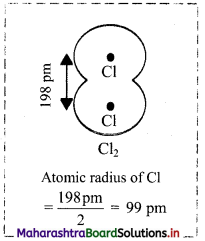Question 54.
One half of the distance between the centres of nucleus of the two adjacent atoms of a metallic crystal is called as a metallic radius.Question 55.
How is metallic radius determined in the case of metals? Give suitable example.

• In the case of metals, distance between the adjacent atoms in metallic sample is measured. One half of this distance is taken as the metallic radius.
• For example: In beryllium, distance between the adjacent Be atoms is measured. One half of this distance is taken as the metallic radius of a Be atom.
• Distance between two adjacent Be atoms is 224 pm. Therefore, metallic radius of a Be atom is 112 pm.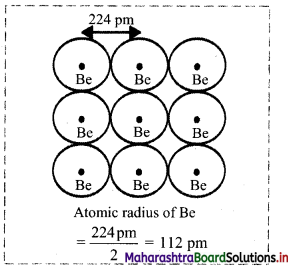Note: Atomic radii of some elements are given in the table below.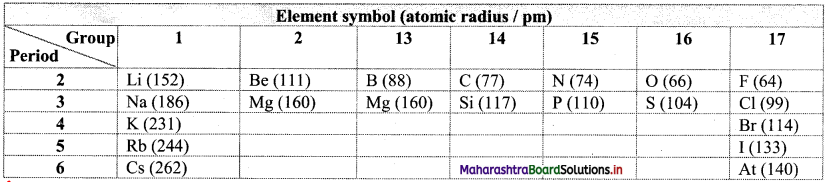Question 56.
How is a cation and an anion formed?
A cation (or positively charged ion) is formed by the removal of one or more electrons from the atom of an element whereas an anion (or negatively charged ion) is formed when the atom of an element gains one or more electrons.

Question 57.
Give reasons: Radius of a cation is smaller and that of an anion is larger as compared to that of their parent atoms.

• A cation is formed by the loss of one or more electrons, therefore, it contains fewer electrons that the parent atom but has the same nuclear charge.
• As a result, the shielding effect is less and effective nuclear charge is larger within a cation. Thus, radius of a cation is smaller than the parent atom.
• However, an anion is formed by the gain of one or more electrons and therefore, it contains a greater number of electrons than the parent atom.
• Due to these additional electrons, anion experiences increased electronic repulsion and decreased effective nuclear charge. As a result, an anion has larger radius than its parent atom.

Hence, radius of a cation is smaller and that of an anion are larger as compared to that of their parent atoms.

Question 58.
Define: Isoelectronic species
The atoms or ions which have the same number of electrons are called isoelectronic species.

Question 59.
Explain with example why the radii of isoelectronic species vary.
i. The radii of isoelectronic species vary according to actual nuclear charge. Larger nuclear charge exerts greater attraction on the electrons and thus, the radius of that isoelectronic species becomes smaller.
ii. For example, F and Na+ both have 10 electrons but the nuclear charge on F is +9 which is smaller than that of Na+ which has the nuclear charge +11.
Hence, F has larger ionic radii (133 pm) than Na+ (98 pm).Question 60.
What is the trend observed in the ionic size of the following isoelectronic species? Explain.
i. Na+, Mg2+, Al3+ and Si4+
ii. O2-, F, Na+ and Mg2+
i. Na+, Mg2+, Al3+ and Si4+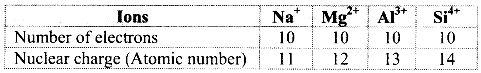a. Among the given ions, the nuclear charge varies but the number of electrons remains the same and therefore, these are isoelectronic species.
b. The radii of isoelectronic species vary according to actual nuclear charge. Larger nuclear charge exerts greater attraction on the electrons and thus, the radius of that isoelectronic species becomes smaller.
c. The nuclear charge increases in the order Na+ < Mg2+ < Al3+ < Si4+ and thus, the ionic size decreases in the order Na+ > Mg2+ > Al3+ > Si4+.

ii. O2-, F, Na+ and Mg2+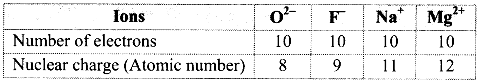a. Among the given ions, the nuclear charge varies but the number of electrons remains the same and therefore, these are isoelectronic species.
b. The radii of isoelectronic species vary according to actual nuclear charge. Larger nuclear charge exerts greater attraction on the electrons and thus, the radius of that isoelectronic species becomes smaller.
c. The nuclear charge increases in the order O2- < F < Na+ < Mg2+ and thus, the ionic size decreases in the order O2- > F > Na+ > Mg2+.

Question 61.
Identify the species having larger radius from the following pairs:
i. Na and Na+
ii. Na+ and Mg2+
i. The nuclear charge is the same in Na and Na+. But Na+ has a smaller number of electrons and a smaller number of occupied shells (two shells in Na+, while three shells in Na). Therefore, radius of Na is larger.
ii. Na+ and Mg2+ are isoelectronic species. Mg2+ has a larger nuclear charge than that of Na+. Therefore, Na+ has larger radius.

Question 62.
Which of the following species will have the largest and the smallest size? Why?
Mg, Mg2+, Al, Al3+

• Atomic radius decreases across the period. Hence, the atomic radius of Mg is larger than that of Al.
• Parent atoms have larger radius than their corresponding cations. Hence, the radius of Mg is larger than that of Mg2+ and the radius of Al is larger than that of Al3+.
• Mg2+ and Al3+ are isoelectronic. Among isoelectronic species, the one with larger nuclear charge will have smaller radius. Al3+ (Z = 13) has a larger nuclear charge than that of Mg2+ (Z = 12). Hence, the ionic radius of Al3+ is smaller than Mg2+.
• Therefore, the decreasing order of radius is Mg > Al > Mg2+ > Al3+.

Hence, species with the largest size is Mg and with the smallest size is Al3+.

Question 63.
Identify the element with more negative value of electron gain enthalpy from the following pairs. Justify.
i. Cl and Br
ii. F and O
i. Cl and Br belong to the same group of halogens with Br having higher atomic number than CL As the atomic number increases down the group, the effective nuclear charge decreases. The increased shielding effect of core electrons can be noticed. The electron has to be added to a farther shell, which releases less energy and thus, electron gain enthalpy becomes less negative down the group. Therefore, Cl has more negative electron gain enthalpy than Br.

ii. F and O belong to the same second period with F having higher atomic number than O. As the atomic number increases across a period, atomic radius decreases, effective nuclear charge increases and electron can be added more easily. Therefore, more energy is released with gain of an electron as we move towards right in a period. Therefore, F has more negative electron gain enthalpy than O.Question 64.
Explain the importance of electronegativity.

• When two atoms of different elements form a covalent bond, the electron pair is shared unequally.
• Electronegativity represents attractive force exerted by the nucleus on shared electrons. Electron sharing between covalently bonded atoms takes place using the valence electron.
• It depends upon the effective nuclear charge experienced by electron involved in formation of the covalent bond.
• Electronegativity predicts the nature of the bond, or, how strong is the force of attraction that holds two atoms together.

Question 65.
Explain the trend in electronegativity
i. across a period
ii. down a group
i. Across a period:
a. As we move across a period from left to right in the periodic table, the effective nuclear charge increases steadily.
b. Hence, due to the increase in effective nuclear charge, the tendency to attract shared electron pair in a covalent bond increases i.e., electronegativity increases from left to right across a period.
e. g. Li < Be < B < C < N < O <F.

ii. Down a group:
a. As we move down the group from top to bottom in the periodic table, the size of the valence shell goes on increasing.
b. However, the effective nuclear charge decreases as the shielding effect of the core electrons increases due to the increase in the size of the atoms.
c. Thus, the tendency to attract shared electron pair in a covalent bond decreases, decreasing the electronegativity down the group.
e.g. F > Cl > Br > I > At.

Question 66.
Explain the terms:
i. Valency of an element
ii. Oxidation state (or oxidation number)
iii. Chemical reactivity
i. Valency of an element:

• Valency of an element indicates the number of chemical bonds that the atom can form giving a molecule.
• The most fundamental chemical property of an element is its combining power. This property is numerically expressed in terms of valency or valence.
• Valence does not have any sign associated with it.
• Valency of the main group elements is usually equal to the number of valence electrons (outer electrons) and/or equal to difference between 8 and the number of valence electrons.

ii. Oxidation state (or oxidation number):

• The oxidation state or oxidation number is a frequently used term related to valence.
• Oxidation number has a sign, + or – which is decided by the electronegativities of atoms that are bonded.

iii. Chemical reactivity:

• Chemical reactivity is related to the ease with which an element loses or gains the electrons.
• Chemical properties of elements depend on their electronic configuration.

Question 67.
What is the trend observed in the valency of main group elements?
i. Valency of the main group elements is usually equal to the number of valence electrons (outer electrons) or it is equal to the difference between 8 and the number of valence electrons.
i.e., (8 – number of valence electrons).
ii. The valency remains the same down the group and shows a gradual variation across the period as atomic number increases from left to right.

Note: Periodic trends in valency of main group elements.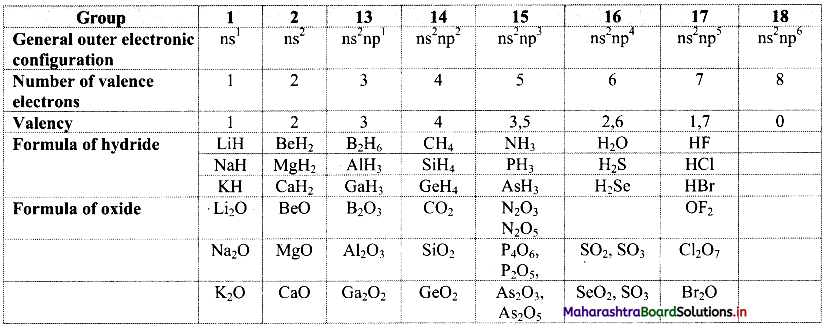Question 68.
Give any two distinguishing points between metals and nonmetals.
Metals:

1. Generally, metals exhibit good electrical conductivity.
2. They can form compounds by loss of valence electrons.

Nonmetals:

1. Generally, nonmetals exhibit poor electrical conductivity.
2. Nonmetals can form compounds by gain of valence electrons in valence shell.Question 69.
Explain the variation of the following property of elements down a group and across a period.
i. Metallic character
ii. Nonmetallic character
The variation observed in the metallic and nonmetallic character of elements can be explained in the terms of ionization enthalpy and electron gain enthalpy.
i. Metallic character:

• The ionization enthalpy decreases down the group. Thus, the tendency to lose valence electrons increases down the group and the metallic character increases down a group.
• However, the ionization enthalpy increases across the period and as a result metallic character decreases across a period.

ii. Nonmetallic character:

• Electron gain enthalpy becomes less negative as we move down the group and hence, nonmetallic character decreases down the group.
• However, electron gain enthalpy becomes more and more negative across the period and thus, nonmetallic character increases across the period.

Question 70.
Justify the position of most reactive and least reactive elements in the modern periodic table.

• Chemical reactivity of elements depends on the ease with which it attains electronic configuration of the nearest inert gas by gaining or losing electrons.
• The elements preceding an inert gas react by gaining electrons in the outermost shell, whereas the elements which follow an inert gas in the periodic table react by loss of valence electrons. Thus, the chemical reactivity is decided by the electron gain enthalpy and ionization enthalpy values, which in turn, are decided by effective nuclear charge and finally by the atomic size.
• The ionization enthalpy is the smallest for the element on the extreme left in a period, whereas the electron gain enthalpy is the most negative for the second last element on the extreme right, (preceding to the inert gas which is the last element of a period).
• Thus, the most reactive elements lie on the extreme left and the extreme right (excluding inert gases) of the periodic table.

Question 71.
How can we predict chemical reactivity of elements based on their oxide formation reactions and the nature of oxides formed?

• The chemical reactivity can be illustrated by comparing the reaction of elements with oxygen to form oxides and the nature of the oxides.
• Alkali metals present on the extreme left of the modem periodic table are highly reactive and thus, they react vigorously with oxygen to form oxides such as Na2O which reacts with water to form strong bases like NaOH.
• The reactive elements on the right i.e., halogens react with oxygen to form oxides such as Cl2O7 which on reaction with water form strong acids like HClO4.
• The oxides of the elements in the centre of the main group elements are either amphoteric (Al2O3) neutral (CO, NO) or weakly acidic (CO2).

Question 72.
Write the chemical equations for reaction, if any, of (i) Na2O and (ii) Al2O3 with HCl and NaOH both. Correlate this with the position of Na and Al in the periodic table, and infer whether the oxides are basic, acidic or amphoteric.
i. Na2O + 2HCl → 2NaCl + H2O
Na2O + NaOH → No reaction
As Na2O reacts with an acid to form salt and water it is a basic oxide. This is because Na is a reactive metal lying on the extreme left of the periodic table.

ii. Al2O3 + 6HCl → 2AlCl3 + 3H2O
Al2O3 + 2NaOH → 2NaAlO2 + H2O
As Al2O3 reacts with an acid as well as base to form a salt and water. It is an amphoteric oxide. Al is a moderately reactive element lying in the centre of main group elements in the periodic table.Question 73.
Comment on the chemical reactivity of d-block and f-block elements.

• d-block (transition) elements and f-block (inner transition) elements exhibit very small change in atomic radii.
• Therefore, the transition and inner transition elements belonging to the individual series have similar chemical properties.
• Their ionization enthalpies are intermediate between those of s-block and p-block elements. Thus, d-block and f-block elements generally show moderate reactivity.

Question 74.
Ge, S and Br belong to the groups 14, 16 and 17, respectively. Predict the empirical formulae of the compounds those can be formed by (i) Ge and S, (ii) Ge and Br.
From the group number we understand that the general outer electronic configuration and number of valence electrons and valencies of the three elements are: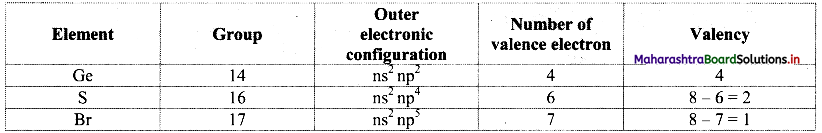i. S is more electronegative than Ge. Therefore, the empirical formula of the compound formed by these two elements is predicted by the method of cross multiplication of the valencies: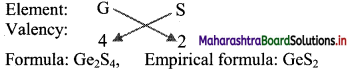ii. Br is more electronegative than Ge. The empirical formula of the compound formed by these two elements is predicted by the method of cross multiplication of valencies: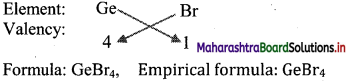[Note: More electronegative element is written on right hand side in cross multiplication method.]

Question 75.
The first ionization enthalpies of 5 elements of second period are given below:

 Element 1st IE values (kJ mol-1) I 520 II 1681 III 1086 IV 2080 V 899

Based on the above data, answer the following questions:
i. Identify the element having highest atomic number.
ii. If element I is lithium, how will you explain its low value of first ionization enthalpy?
iii. Explain why ionization enthalpies are always positive.
i. Element IV. The first ionization enthalpy increases with increase in atomic number along a period. Hence, the element IV having highest IE will have highest atomic number among the given elements.
ii. Alkali metals have only one electron in their valence shell which can be easily lost resulting in the stable noble gas configuration. Therefore, lithium shows low value of first ionization enthalpy.
iii. Energy is always required to remove electrons from an atom. Hence, ionization enthalpies have positive value.

Question 76.
From the elements Mg, Ar, Cl, Sr, P and S, choose one that fits each of the below given descriptions:
i. An element having two valence electrons.
ii. An element having properties similar to that of O.
iii. A noble gas.
iv. An alkaline earth metal,
v. An element having electronic configuration 1s22s22p63s23p3.
i. Magnesium (Mg)
ii. Sulphur (S)
iii. Argon (Ar)
iv. Strontium (Sr)
v. Phosphorus (P)

Multiple Choice Questions

1. Mendeleev’s periodic table had …………… elements.
(A) 75
(B) 83
(C) 63
(D) 118
(C) 63

2. The serial or ordinal number of an element in Mendeleev’s periodic table was recognized as ………….
(A) neutron number
(B) valency
(C) principal quantum number
(D) proton number
(D) proton number3. Mendeleev predicted the existence of …………..
(A) aluminium
(B) silicon
(C) tellurium
(D) germanium
(D) germanium

4. According to Mendeleev’s periodic law, the physical and chemical properties of elements are the periodic function of their …………..
(A) atomic weights
(B) atomic numbers
(C) molecular formulas
(D) molecular weights
(A) atomic weights

5. Moseley showed that the fundamental property of an element is ……………
(A) atomic number
(B) atomic mass
(C) both A and B
(D) none of these
(A) atomic number

6. According to periodic law of elements, the variation in properties of elements is related to their ……………
(A) densities
(B) atomic masses
(C) atomic sizes
(D) atomic numbers
(D) atomic numbers

7. At present, how many elements are known?
(A) 118
(B) 110
(C) 114
(D) 120
(A) 1188. The long form of the periodic table consists of how many periods?
(A) 5
(B) 8
(C) 10
(D) 7
(D) 7

9. According to quantum mechanical model of the atom, the properties of elements can be correlated to their …………….
(A) atomic number
(B) atomic mass
(C) valency
(D) electronic configuration
(D) electronic configuration

10. The fourth, fifth and sixth periods are long periods and contain ……………
(A) 18, 18 and 36
(B) 18, 28 and 32
(C) 18, 15 and 31
(D) 18, 18 and 32
(D) 18, 18 and 32

11. f-block elements are also known as ……………
(A) transition elements
(B) inert gas elements
(C) normal elements
(D) inner transition elements
(D) inner transition elements

12. Which of the following forms a bridge between reactive s-block elements and less reactive group 13 and 14 elements?
(A) Inert gases
(B) Transition metals
(C) Halogens
(D) Inner transition metals
(B) Transition metals

13. ………… elements are known as chalcogens.
(A) Group 17
(B) Group 18
(C) Group 16
(D) Group 1
(C) Group 1614. The name ‘rare earth elements’ is used for …………..
(A) lanthanides only
(B) actinides only
(C) both lanthanides and actinides
(D) alkaline earth metals
(C) both lanthanides and actinides

15. Atomic number of V is 23 and its electronic configuration is …………….
(A) 1s2 2s2 2p6 3p6 3d3 4s2
(B) 1s2 2s2 2d3 3p6 2p6 4s2
(C) 2s2 1s2 2p6 3s2 3d3 4s2
(D) 1s2 2s2 2p6 3s2 3p6 3d3 4s2
(D) 1s2 2s2 2p6 3s2 3p6 3d3 4s2

16. Aluminium belongs to …………. elements.
(A) s-block
(B) p-block
(C) d-block
(D) f-block
(B) p-block

17. In P3-, S2- and Cl ions, the increasing order of size is ………….
(A) Cl < S2- < P3-
(B) P3- < S2- < Cl
(C) S2- < Cl < P3-
(D) S2- < P3- < Cl
(A) Cl- < S2- < P3-

18. The CORRECT order of radii is ……………
(A) N < Be < B
(B) F< O2- <N3-
(C) Na < Li < K
(D) Fe3+ < Fe2+ < Fe4+
(B) F < O2- <N3-19. Which of the following species will have the largest size Mg, Mg2+, Fe, Fe3+?
(A) Mg
(B) Mg2+
(C) Fe
(D) Fe3+
(C) Fe

20. Which one of the following is CORRECT order of the size?
(A) I > I >I+
(B) I > I+ > I
(C) I+ > I > I
(D) I > I > I+
(D) I > I > I+

21. The CORRECT order of increasing radii of the elements Na, Si, Al and P is ……………
(A) Si < Al < P < Na
(B) Al < Si < P < Na
(C) P < Si < Al < Na
(D) Al < P < Si < Na
(C) P < Si < Al < Na

22. The metallic and nonmetallic properties of elements can be judged by their ……………
(A) electron gain enthalpy
(B) ionization enthalpy
(C) electronegativity
(D) valence
(C) electronegativity23. Which element has the most negative electron gain enthalpy?
(A) Sulphur
(B) Fluorine
(C) Chlorine
(D) Hydrogen